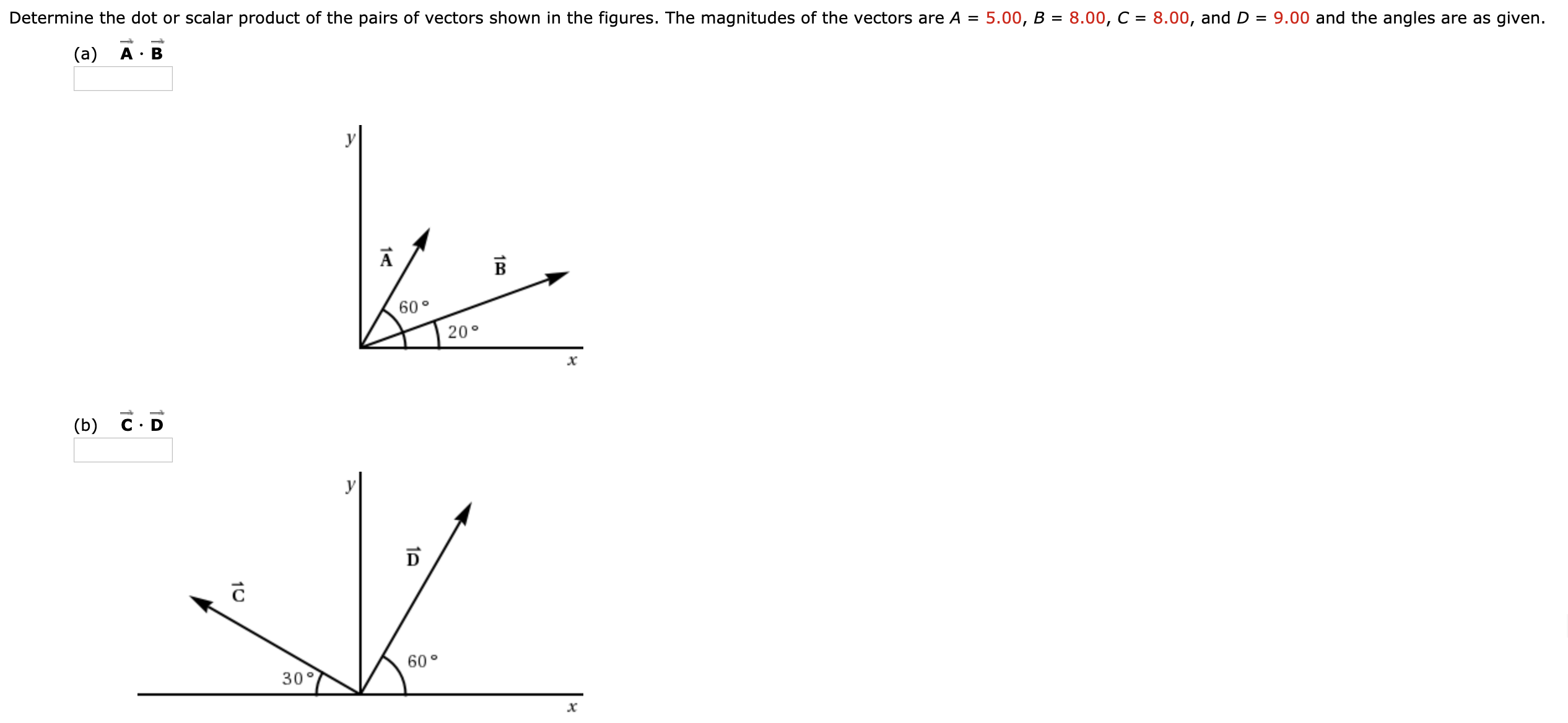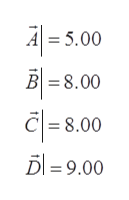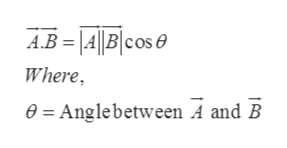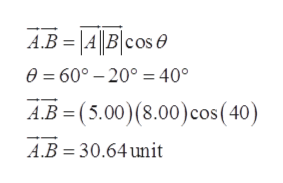9.00 and the angles are as givenDetermine the dot or scalar product of the pairs of vectors shown in the figures. The magnitudes of the vectors are A =5.00, B8.00, C8.00, and D(a)B6020°(b) C Dy60°30°1001A14C10

Question

Please show me how to work this. Don't round any figures please.help_outlineImage Transcriptionclose9.00 and the angles are as given Determine the dot or scalar product of the pairs of vectors shown in the figures. The magnitudes of the vectors are A = 5.00, B 8.00, C 8.00, and D (a) B 60 20° (b) C D y 60° 30° 100 1A 14C 10 fullscreen
Step 1

The dot product of two vectors can be calculated using following steps,

Given:

The magnitude of vectors is,help_outlineImage TranscriptioncloseA = 5.00 B 8.00 = 8.00 D =9.00 fullscreen
Step 2

Calculation:

The scalar product of two vectors is given by,help_outlineImage TranscriptioncloseABA|B|cose Where e= Anglebetween A and B fullscreen
Step 3

Part (a):

The scalar product ...help_outlineImage TranscriptioncloseА.В - |4|B/сos @ e=60°-200 40° A.B (5.00) (8.00) cos (40) A.B 30.64 unit fullscreen

Want to see the full answer?

See Solution

Want to see this answer and more?

Our solutions are written by experts, many with advanced degrees, and available 24/7

See Solution
Tagged in

Vectors and Scalars